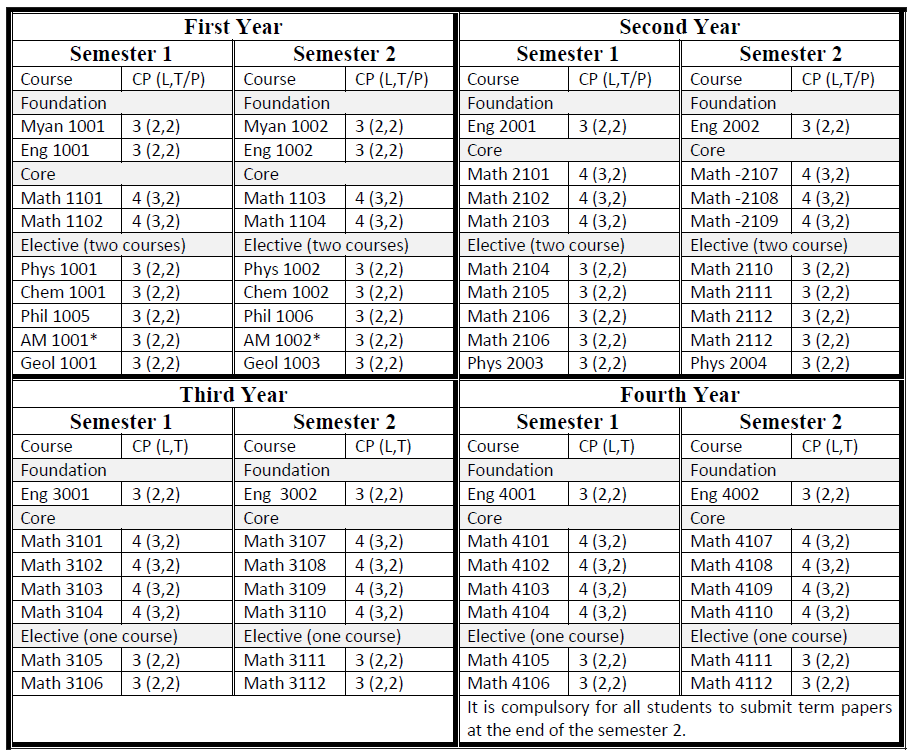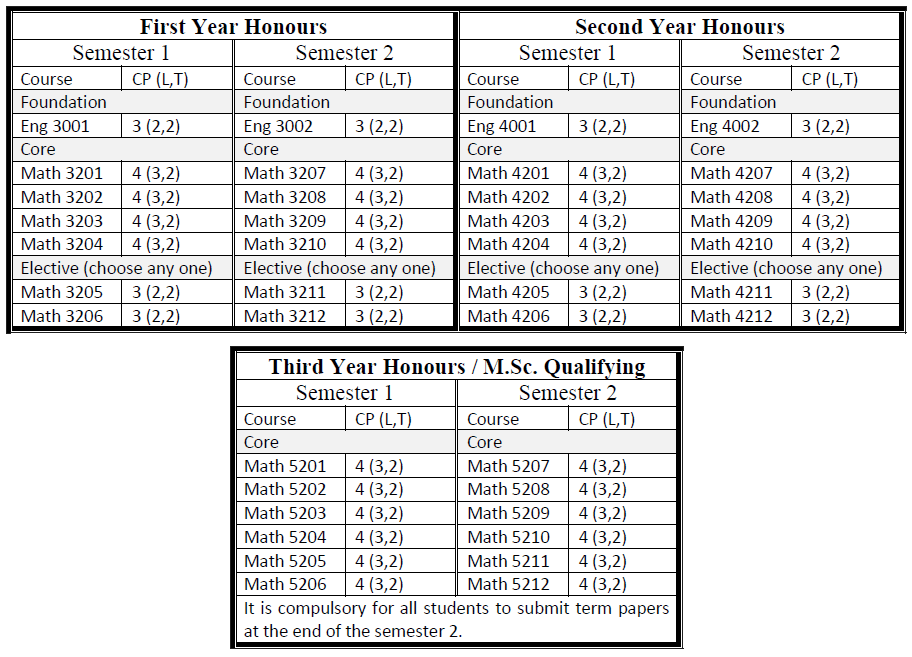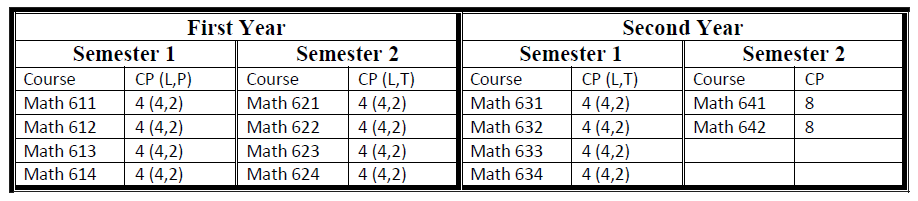### Curriculum

#### B.Sc. in Mathematics#### B.Sc. (Honours) in Mathematics

Students who passed second year with GPA ≥4 are eligible to attend B.Sc. (Honours) classes for three more years. After finished successfully, they are earned B.Sc. (Hons) degree majoring in Mathematics.#### M.Sc. in Mathematics### Course Descriptions

#### Math 1101: Algebra and Analytic Geometry

Algebra: logic and sets, polynomial functions, permutations, combinations, binomial theorem, mathematical induction.
Analytic Geometry: lines, circles, conic sections, translation of coordinate axes, rotation of coordinate axes.

#### Math 1102: Trigonometry and Differential Calculus

Trigonometry: inverse trigonometric functions
Calculus: limits, continuity exponential, logarithmic, hyperbolic functions and their inverse, differentiation of inverse trigonometric functions, differentiation of exponential, L’Hopital’s rule, Taylor series.

#### Math 1103: Algebra and Analytical Solid Geometry

Algebra: determinants, matrices, complex numbers. Polar Coordinates System: polar coordinates, graphing in polar coordinates, areas and lengths in polar coordinates.
Analytical Solid Geometry: three dimensional Cartesian coordinate system, lines, planes, quadric surfaces.

#### Math 1104: Differential and Integral Calculus

Extreme values of functions, the mean value theorem, monotonic functions and the first derivatives test, methods of integration, improper integrals, applications of integration, partial differentiation, ordinary differential equation of first order.

#### Math 2101: Complex Variables I

Analytic functions, elementary functions, integrals, residues and poles.

#### Math 2102: Calculus of Several Variables

Functions of two or more variables, partial derivatives, directional derivatives, chain rule for partial derivatives, total differential, maxima and minima, exact differentials, derivatives of integrals, double integrals in Cartesian coordinates and polar coordinates, triple integrals in Cartesian coordinates, cylindrical coordinates and spherical coordinates, applications of multiple integrals.

#### Math 2103: Vector Algebra and Statics

Vector Algebra: dot products, cross products, triple scalar products, triple vector products.
Statics: statics of a particle, tension of a string, friction, moment and couples, centre of gravity, statics of a rigid body in a plane, jointed rods, virtual works and stability.

#### Math 2104: Discrete Mathematics I

Counting Methods and the Pigeonhole Principle: basic principle, permutations and combinations; algorithms for generating permutations and combinations; generalized permutations and combinations; binomial coefficients and combinatorial identities; and the pigeonhole principle.

#### Math 2105: Theory of Sets I

Cardinal numbers, partially and totally ordered sets.

#### Math 2106: Spherical Trigonometry and Its Applications

Spherical Trigonometry: the spherical triangle, length of small circle arc, terrestrial latitude and longitude, the fundamental formula of spherical trigonometry, the sine formula, formula C, the four parts formula, the trigonometrical ratios for small angles.
Celestial Sphere: altitude and azimuth, declination and hour angle, diagram for the southern hemisphere, circumpolar stars, the standard or geocentric celestial sphere, right ascension and declination, the earth’s orbits, celestial latitude and longitude, sidereal time, mean solar time, hour angle of a heavenly body, rising and setting, and Twilight.

#### Math 2107: Linear Algebra I

Vector spaces, subspaces, bases and dimensions, sums and direct sums, linear mapping, kernel and image of linear map and their dimension, and compositions of linear mapping.

#### Math 2108: Ordinary Differential Equations

Second-order linear differential equations, higher-order linear differential equations, and system of differential equations.

#### Math 2109: Vector Calculus and Dynamics

Vector Calculus: gradient, divergence, curl, line integrals, green’s theorem, divergence theorem, Stoke’s theorem.
Dynamics: kinematics of a particle, relative velocity, mass, momentum and force, Newton’s law of motion, work, power and energy, impulsive force, projectiles, simple harmonic motions, kinematics of a particle in two dimensions, and kinetic of a particle in two dimensions.

#### Math 2110: Discrete Mathematics II

Graph Theory: introduction, paths and cycles, Hamiltonian cycles and the traveling salesperson problem, a shortest path algorithm, representations of graphs, isomorphism of graphs, planar graphs.
Trees: introduction, terminology and characterizations of trees, spanning trees, minimal spanning trees, binary trees.

#### Math 2111: Theory of Sets II

Well-ordered sets, ordinal numbers, axiom of choice, Zorn’s lemma, well-ordering theorem.

#### Math 2112: Astronomy

Planetary Motions: Kepler’s three laws, Newton’s law of gravitation, the masses of the planets, the dynamical principles of orbital motion, the equation of the orbit, velocity of a planet in its orbit, components of the linear velocity perpendicular to radius vector and to the major axis, the true and eccentric anomaly expressed as a series in terms of e and the eccentric anomaly, the equation of the centre, the orbit in the space, the orbital and synodic periods of a planet, the earth’s orbit, the sun’s orbit, the moon’s orbit.
Time: sidereal time, the mean sun, the sidereal year and the tropical year, relation between mean and sidereal time, the calendar, the Julian date, the equation of time, the seasons.

#### Math 3101/ 3201: Analysis I

Elements of set theory, numerical sequences and series.

#### Math 3102/ 3202: Linear Algebra II

Linear maps and matrices, and determinants.

#### Math 3103/ 3203: Differential Equations

Series solutions of differential equations, special functions, and laplace transforms.

#### Math 3104/ 3204: Differential Geometry

Concept of a curve, curvature and torsion, the theory of curves, and concept of a surface.

#### Math 3105/ 3205: Tensor Analysis

Curvilinear coordinates, and tensor analysis

#### Math 3106/ 3206: Number Theory I

Divisibility theory, congruences, Fermat’s little theorem, Euler’s generalization of FLT, Wilson’s theorem, and Eulers-function.

#### Math 3107/ 3207: Analysis II

Continuity and differentiation

#### Math 3108/ 3208: Linear Algebra III

Scalar products and orthogonality, matrices and bilinear maps

#### Math 3109/ 3209: Mechanics

Central force motion, kinematics of plane rigid bodies, kinetics of plane rigid bodies, impact, dynamics of a particle in three dimensions, dynamics of system of particles, and moment of inertia.

#### Math 3110/ 3210: Probability and Statistics

Introduction to probability theory, random variables, conditional probability and conditional expectation, mean, median, mode, standard deviation, correlation, and regression.

#### Math 3111/ 3211: Complex Variables II

Conformal mapping and application of conformal mapping.

#### Math 3112/ 3212: Number Theory II

Primitive roots, quadratic congruence and quadratic reciprocity law, perfect numbers and Fermat’s numbers, and representation of integers as sum of squares.

#### Math 4101/ 4201: Analysis III

Methods of mathematical research and the Riemann-Stieltjes integral.

#### Math 4102/ 4202: Numerical Analysis I

Numerical methods in general and numerical methods in linear algebra.

#### Math 4103/ 4203: Linear Programming

Basic properties of linear programs, the simplex method, duality, dual simplex method and primal dual algorithms.

#### Math 4104/ 4204: Partial Differential Equations

Fourier series, integrals and transforms, and partial differential equations.

Markov chains

#### Math 4106/ 4206: Fundamentals of Algorithms and Computer Programming

The idea of an algorithm, pseudo code descriptions of algorithms, efficiency of algorithms, algorithms for arithmetic and algebra, coding and implementations of algorithms in some programming languages.

#### Math 4107/ 4207: Analysis IV

Sequences and series of functions

#### Math 4108/ 4208: General Topology I

Topology of the line and plane, topological spaces, bases and sub-bases.

#### Math 4109/ 4209: Abstract Algebra I

Semigroups, monoids and groups, homomorphisms and subgroups, cyclic groups, cosets and counting, normality, quotient groups, and homomorphisms.

#### Math 4110/ 4210: Hydromechanics

Density and specific gravity, theorems on fluid-pressure under gravity, pressure of heavy fluids, thrusts on curved surfaces and floating bodies, stability of floating bodies, equation of continuity, equation of motion, and some three dimensional flows.

#### Math 4111/ 4211: Stochastic Process II

The exponential distribution and the Poisson process.

#### Math 4112/ 4212: Integer Programming

Formulations of integer programming, branch and bound algorithms, and cutting plane methods.

#### Math 5201: Analysis V

Power series, the exponential and logarithmic functions, the trigonometric functions, and Fourier series.

#### Math 5202: General Topology II

Continuity and topological equivalence, metric and normed spaces

#### Math 5203: Abstract Algebra II

Symmetric, alternating, and dihedral groups, categories, products, coproducts, and free objects, direct products and direct sums, free groups, free products, generators and relations.

#### Math 5204: Hydrodynamics I

Axisymmetric flow, Stoke’s stream function, some two-dimensional flows, and general motion of cylinder

#### Math 5205: Numerical Analysis II

Numerical methods in linear algebra, and numerical methods for differential equations.

#### Math 5206: Qualitative Theory of Ordinary Differential Equations I

Systems of differential equations, and linear systems with an introduction to phase space analysis.

#### Math 5207: Analysis VI

Continuous transformations of metric spaces, Euclidean spaces, continuous functions of several real variables, partial derivatives, linear transformations and determinants, the inverse function theorem, the implicit function theorem, functional dependence.

#### Math 5208: General Topology III

Separation axioms, compactness, concept of product topology and examples.

#### Math 5209: Abstract Algebra III

Free abelian groups, finitely generated abelian groups, the Krull-Schmidt theorem, the action of a group on a set, the Sylow theorems.

#### Math 5210: Hydromechanics II

Two-dimensional vortex motion, and water waves

#### Math 5211: Graph Theory

Graphs and subgraphs, trees

#### Math 5212: Qualitative Theory of Ordinary Differential Equations II

Existence theory, stability of linear and almost linear systems

#### Math 611: Algebra I

Group-homomorphism, Group-Isomorphism, Group-Automorphism, Symmetric groups, Lagrange theorem, Quotient groups, Conjugation, p-groups and Sylow theory.

#### Math 612: Analysis I

Normed spaces, Banach spaces, Hilbert spaces, Standard theorems.

#### Math 613: Differential Equations I

Existence and uniqueness of solution of the first order ordinary differential equation, Linear system, Stability, First order quasi-linear partial differential equations, Cauchy problem.

#### Math 614: Applied Mathematics I

(a) Basic equations, Inviscid Two-dimensional flow.
(b) Probability spaces, Mutually exclusive and independent events, Conditional probability, Discrete and continuous random variable, Density function, Distribution function, Expectation and variance, Some useful distribution function, Some useful inequalities, Generating functions and characteristic functions.

#### Math 621: Algebra II

Rings, Ideals, Quotient rings, Ring Homomorphism, Commutative rings, Integral domains, Unique factorization domains, Field, Field extensions.

#### Math 622: Analysis II

Linear Operators, Bounded Operators, Unbounded Operators, Spectral Theory.

#### Math 623: Differential Equations II

Ordinary Differential Equation: Phase space Analysis, Existence Theory, Stability of Nonlinear System.

#### Math 624: Applied Mathematics II

(a) Irrotational motion, Vortex Motion.
(b) Law of large numbers, Order in probability, Poisson process, Branching process, Birth and death process, Random walks.

#### Math 631: Pure Mathematics I

Frechet spaces, Locally convex spaces, Duality, Linear operators.
(a) Compact operators, Fredholm operators, Unbounded operators, Semigroup.

#### Math 632: Mathematical Methods I

(a) Differential Equations and related topics: Theory of Elliptic, Parabolic and Hyperbolic Equations.
(b) Computational Mathematics: Numerical Linear Algebra

#### Math 633: Physical Applied Mathematics I

Viscous Motion: Viscous Incompressible Motion, Equation of Motion, Exact Solution.

#### Math 634: Social Applied Mathematics I

(a) Introduction to mathematical statistics.
(b) Linear Programming: basic concepts, fundamental theorem of linear programming, simplex method, revised simplex method, duality theorem, complementary slackness theorem, sensitivity analysis, dual simplex method, primal dual algorithms, Integer Programming branch and bound algorithms, cutting plane methods.

## Prof. Dr. Hla Hla Kyi

##### Head of Department - Mathematics
Department of Mathematics, Dagon University, Bohmu Ba Htoo Road, Yangon
(+95-01) 585171
mathematics@dagonuniversity.edu.mm

Stay in Touch:
Scroll Up